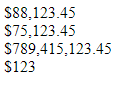Related Articles

# How to format a number as currency using CSS ?

• Last Updated : 03 Jun, 2020

Given a number and the task is to format the number of an HTML element as currency using CSS and a little bit of JavaScript. It is not possible to complete the task with pure CSS. A bit of JavaScript is needed for the parsing of the number to add the commas.

Approach: In the code, the CSS class `currSign` adds the currency sign (like ‘\$’) before the number. The JavaScript function `toLocaleString()` returns a string with a language-sensitive representation of the number.

Hey geek! The constant emerging technologies in the world of web development always keeps the excitement for this subject through the roof. But before you tackle the big projects, we suggest you start by learning the basics. Kickstart your web development journey by learning JS concepts with our JavaScript Course. Now at it's lowest price ever!

Example:

 ```<``html``>`` ` `<``head``>``    ``<``meta` `name``=``"viewport"` `content``=``        ``"width=device-width, initial-scale=1"``>`` ` `    ````    ``<``style``>``        ``.currSign:before {``            ``content: '\$';``        ``}``    `````` ` `<``body``>``    ````    ``<``div` `class``=``"myDIV"``>88123.45``    ``<``div` `class``=``"myDIV"``>75123.45``    ``<``div` `class``=``"myDIV"``>789415123.45``    ``<``div` `class``=``"myDIV"``>123`` ` `    ````    ``<``script``>``        ``let x = document.querySelectorAll(".myDIV");``        ``for (let i = 0, len = x.length; i < ``len``; i++) {``            ``let ``num` `= ``Number``(x[i].innerHTML)``                      ``.toLocaleString('en');``            ``x[i]``.innerHTML` `= ``num``;``            ``x[i].classList.add("currSign");``        ``}``    `````` ` ``

Output:My Personal Notes arrow_drop_up